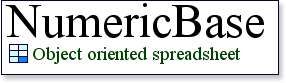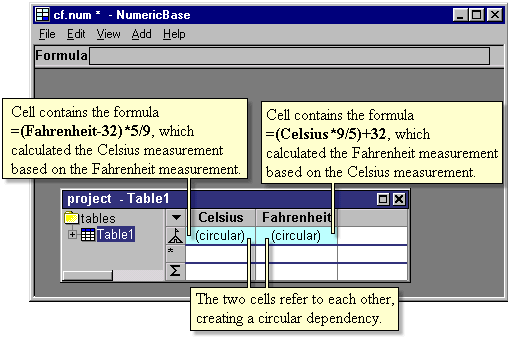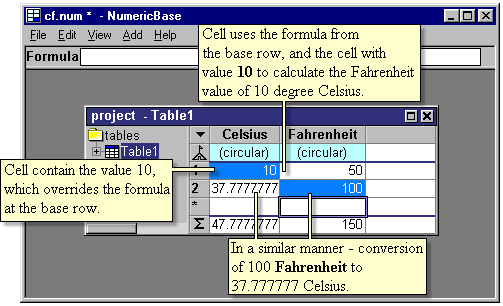# Circular dependency

Circular dependency
NumericBase treats circular dependency not as an error, but as a mean to create better solutions. A circular dependency happens when the formula controlling a cell refers, either directly or indirectly, to the same cell.

When this happens, NumericBase sets the value of the cell be a "circular" message value. No other message or pop up window is displayed to indicate this circular dependency.

Temperature conversion
In the following screenshot, two cells refer to each other to implement temperature conversion between Celsius and Fahrenheit.  Both cells refer to each other, creating a circular dependency.Screenshot: Circular dependency between two cells.

"What you know" modeling
Some problems consist of several values. If you know enough of these values, you can calculate the rest using the relationships that exist among the values. For example, a loan with fixed interest rate and fixed payment consist of four values : interest rate, loan amount, term, and the fixed payment. If you know any 3 of the four values, you can calculate the remaining value.

Another example
Another example is temperature measurement. You can express the temperature in two alternative scales: Celsius or Fahrenheit. If you know the value in one scale, you can calculate the value in the other, using the conversion formulas.

Using Circular dependency to solve "What you know" problems.
NumericBase let you solve "what you know" problems like these, by using circular formulas. You define the circular formulas in the base row, and then override them with the values that you know at the data rows. NumericBase will calculate the rest of the values in the data rows, by using the formulas that you defined in the base row.

Back to temperature conversion
The following screenshot, uses this trick to do some bi-directional temperature conversions. It convert 10 degree Celsius to 50 degree Fahrenheit, and 100 degree Fahrenheit to 37.777777 degrees Celsius.Screenshot: using circular dependency for temperature conversion.

The "alt" operator.
The "alt" operator let you specify several alternative formulas in a cell. NumericBase will use the first formula that does not result in a (circular) message value or an error value.

This is useful to solve "what you know" problems that can not be solved using the method outlined above. For example, elaborating on the last problem, temperature conversion between three scales : Celsius, Fahrenheit, and Kelvin. This can not be solved using the last method alone, but it can be solved using the "alt" operator.

The following screenshot uses the alt operator in the "Celsius" column. The formula there is
=(Fahrenheit-32)*5/9 alt Kelvin-273
The formula  uses the alt operator to specify two different conversion formulas: from Celsius and from Kelvin.

The screenshot shows three conversion from three different scales. These conversions are from 10 degrees Celsius, from 10 degrees Fahrenheit, and from 10 degrees Kelvin.Screenshot: using the alt operator.